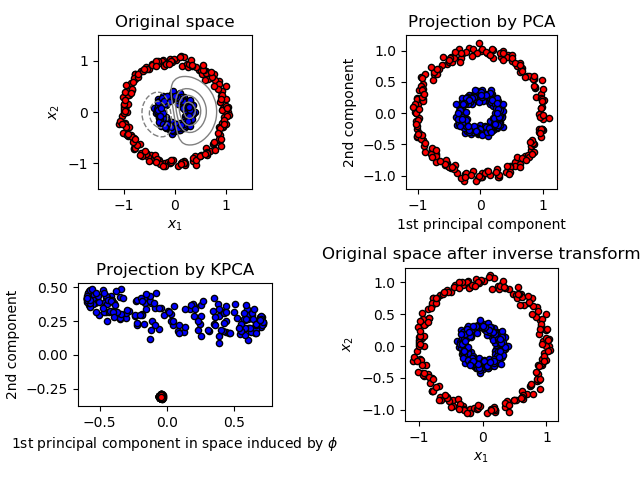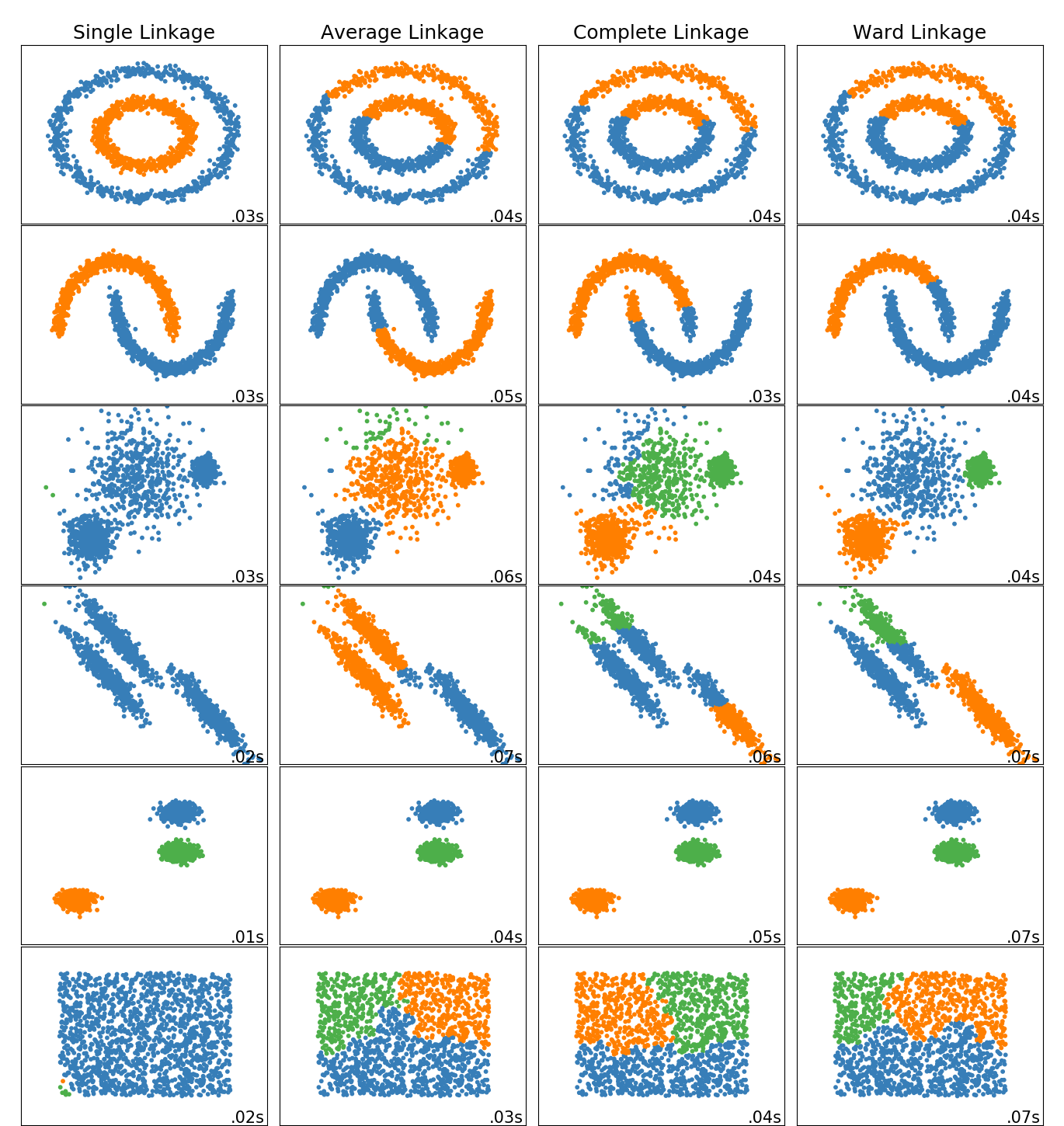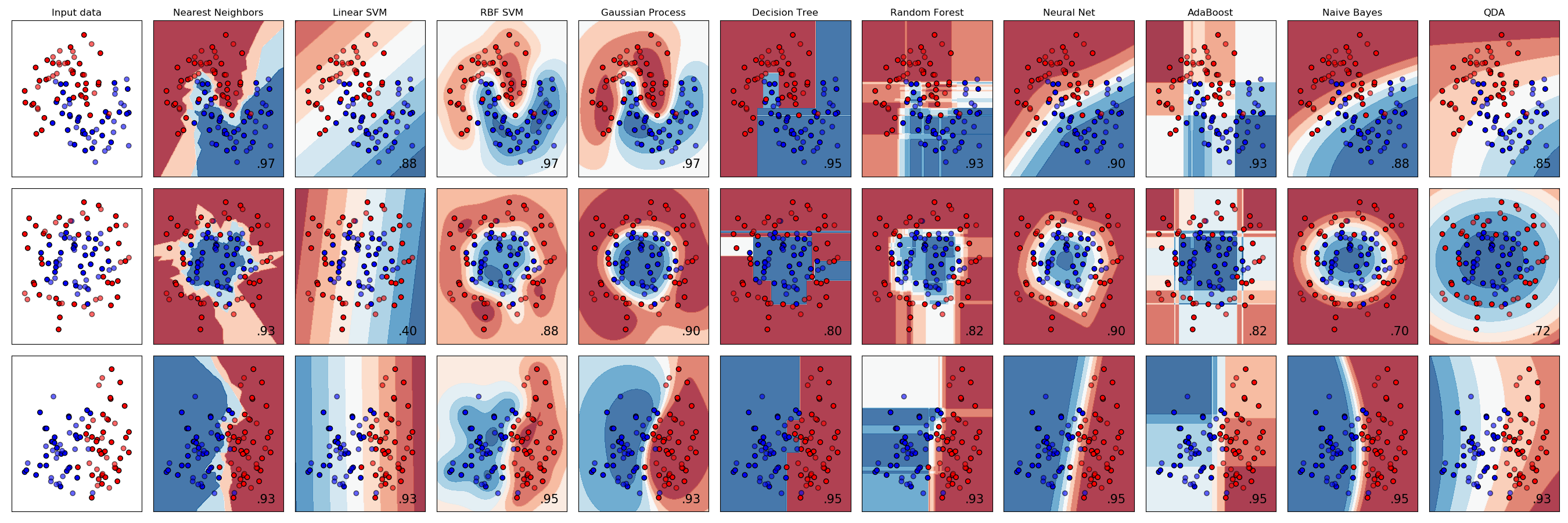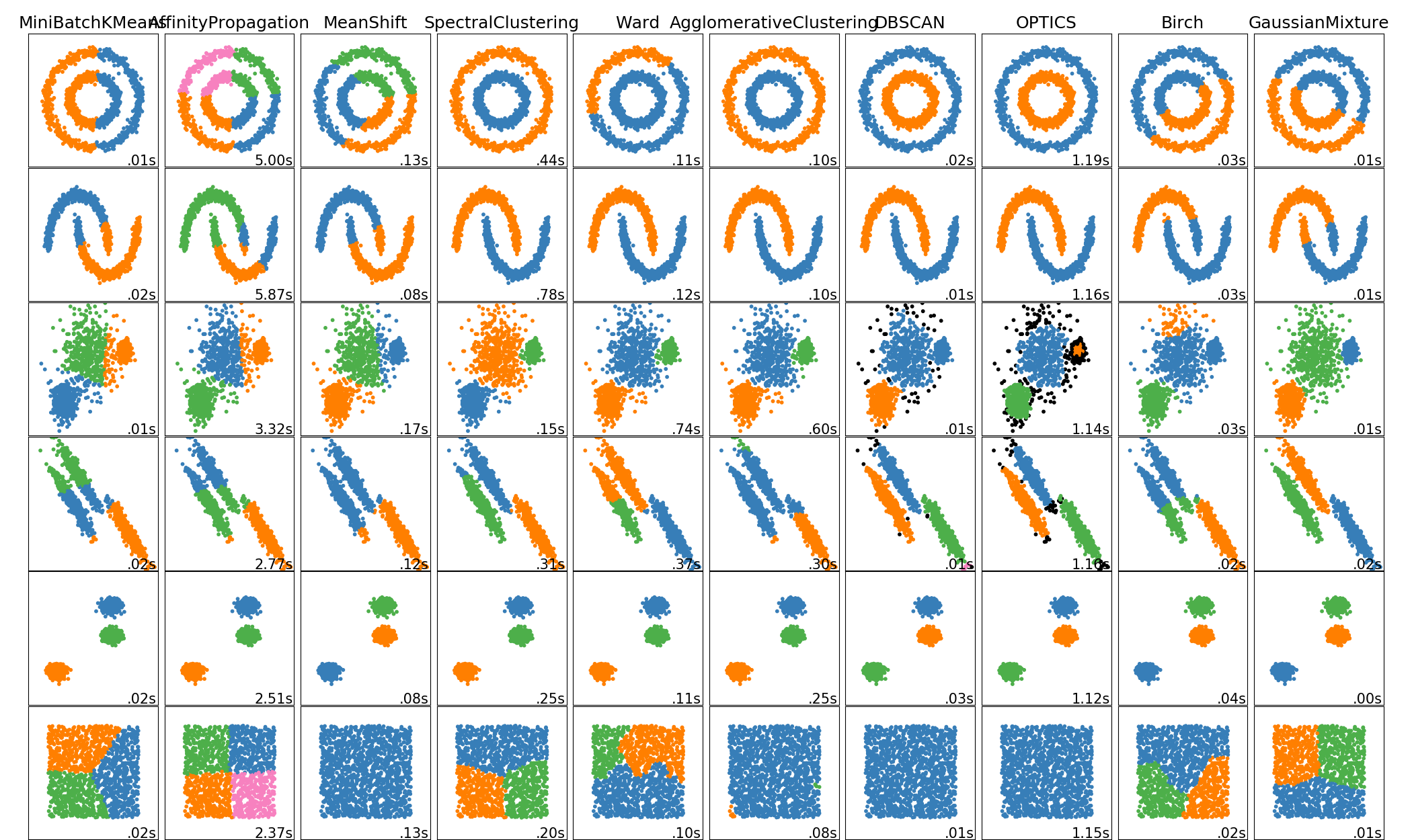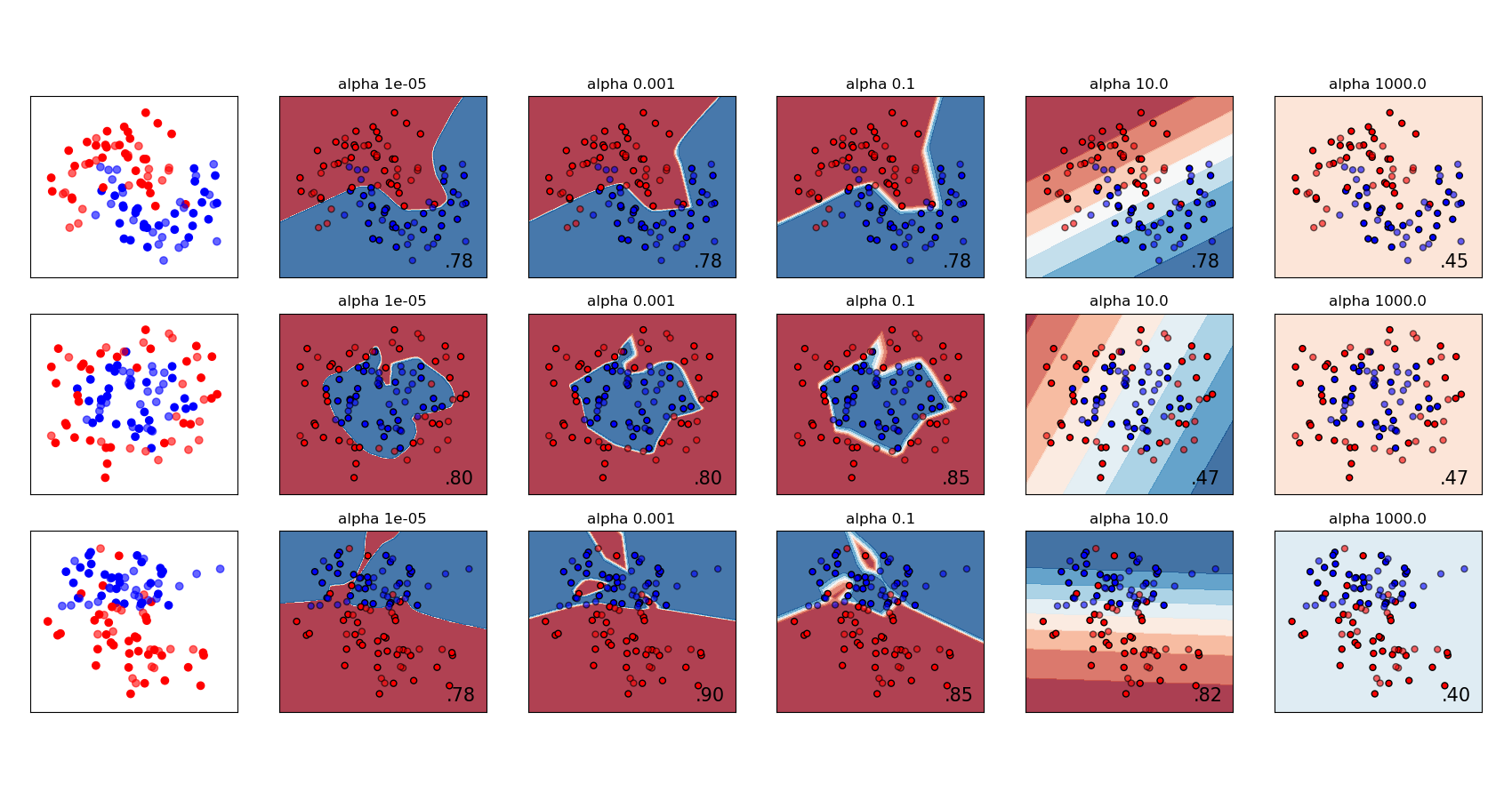# sklearn.datasets.make_circles¶

sklearn.datasets.make_circles(n_samples=100, *, shuffle=True, noise=None, random_state=None, factor=0.8)

[源码]

n_samples int or two-element tuple, optional (default=100)

shuffle bool, optional (default=True)

noise double or None (default=None)

random_state int, RandomState instance, default=None

factor 0 < double < 1 (default=.8)

X array of shape [n_samples, 2]

y array of shape [n_samples]

## sklearn.datasets.make_circles使用示例¶